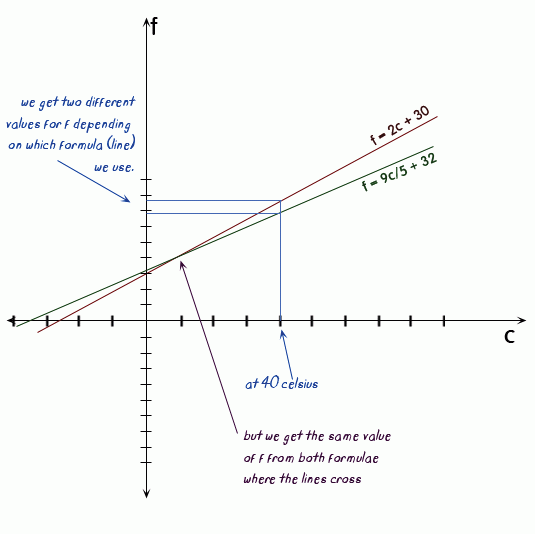SEARCH HOMEMath Central Quandaries & QueriesQuestion from kyrie, a student: help ? temperature formula: f and c represent degree's of temperature. exact formula: f = 9c / 5 + 32 approx formula: f = 2c + 30 at what temp in c do these formulae give an equal value for f ?Hi Kyrie.

If both give the same value for f, then the f values equal each other.
Two things that both equal a third thing have to equal each other, so
9c / 5 + 32 = 2c + 30

Solve for c.

Stephen La Rocque.>

Kyrie wrote me back saying:

hi sue
ref my question you answered yesterday on temperature ?
f = 9c / 5 + 32
f = 2c + 30
your ans was   9c / 5 + 32 = 2c + 30 solve c
is c =  -1.428   if so, temp of f are not equal ? i'm confused ?

Hi Kyrie.

That's right. The two equations are giving you two different values for f for a given value c.

Look at your two equations. They are linear equations (that means when you graph them, each one forms a line). In the spot where the two lines cross, they give the same value of f for a particular value of c. Let's graph them with f as the vertical axis (replaces the usual y axis) and c as the horizontal axis (replaces the usual x axis):So when you solve for c, you are solving for that point of intersection where the two values of f are the same.

9c/5 + 32 = 2c + 30
c/5 = 2
c = 10.

So this tells us that when c is 10 celsius, both formulae give the same value for f. Let's check:

f = 9(10)/5 + 32
f = 18 + 32 = 50.

f = 2(10) + 30
f = 20 + 30 = 50.

Yes this checks out.

If you try it for -1.428, you will find that they give different values, so that is not the answer.

Hope this graph helps you to understand how it works, Kyrie.
Stephen La Rocque.Math Central is supported by the University of Regina and The Pacific Institute for the Mathematical Sciences.